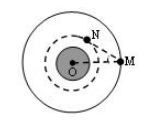O 连线间的夹角最大为θ，则 M、N 的运动周期之比等$\text{A.}$ $\sin ^3 \theta$ $\text{B.}$ $\frac{1}{\sin ^3 \theta}$ $\text{C.}$ $\sqrt{\sin ^3 \theta}$ $\text{D.}$ $\sqrt{\frac{1}{\sin ^3 \theta}}$

D